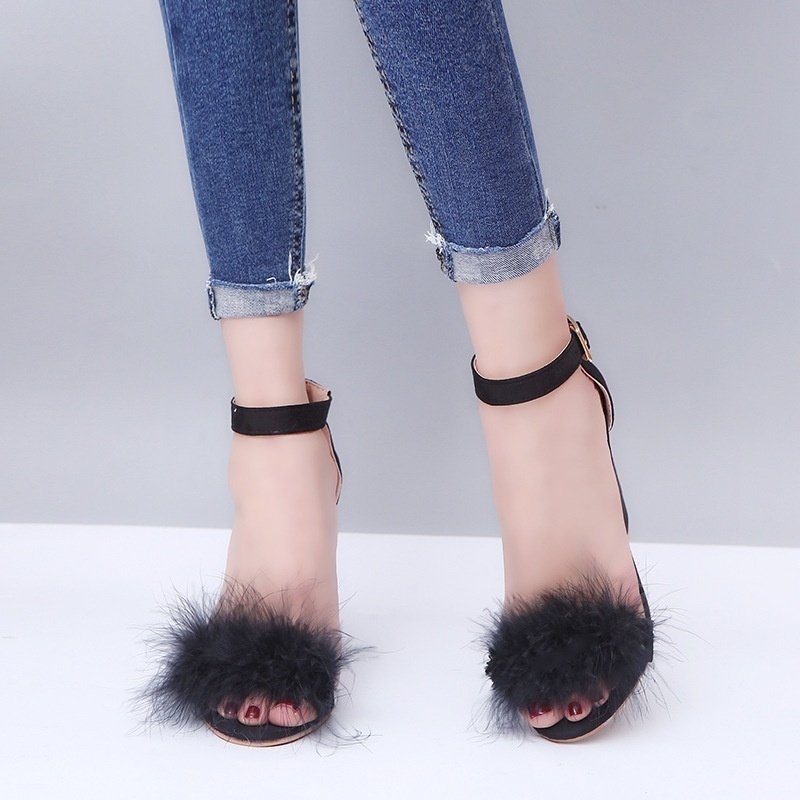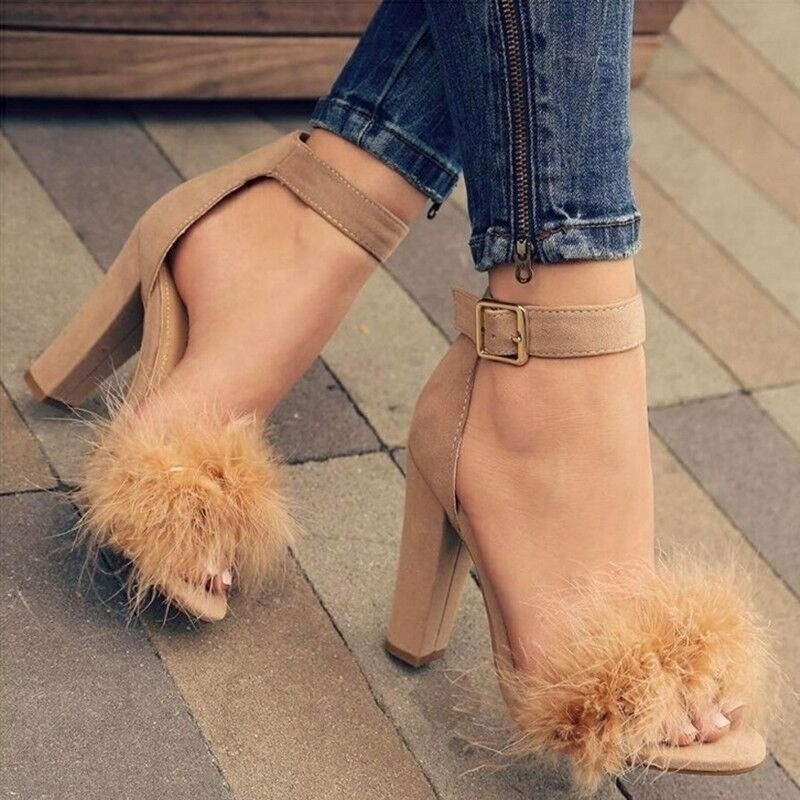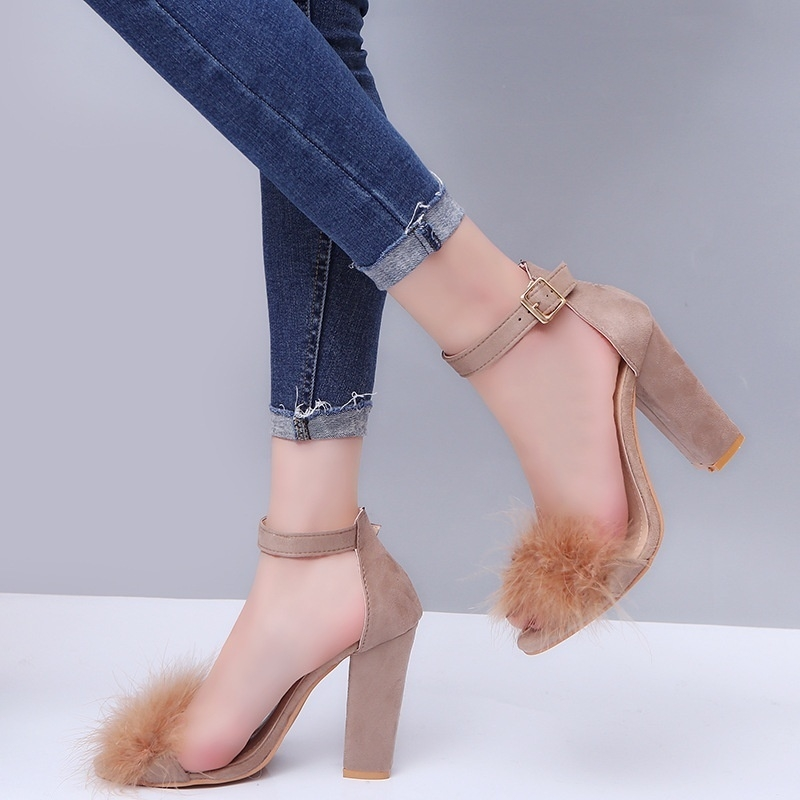# Fashion Stylish Size 35-42 Women Rabbit Hair High-heeled Sandals

5 out of 5
(1 rating)
KSh 2,500
KSh 3,50029%
Select Variation

## Delivery & Returns

### Delivery Information

Delivered between Tuesday 14 Apr and Thursday 16 Apr.See more

### Return Policy

Easy Return, Quick Refund.See more

N/A

## Seller Information

faina fashion n beauty

80%Positive Seller Score

24 Followers

## Product details

• 100%High quality and new brand
• Upper Material:Suede
• Color:brown,black
• Heel Height:above 8cm
• Size:35/36/37/38/39/40/41/42/43
• 35=EU size=35=US size=6=UK size=4=Length(cm)=22.5cm=Length(inch)=8.86inch
• 36=EU size=36=US size=6.5=UK size=4.5=Length(cm)=23cm=Length(inch)=9.06inch
• 37=EU size=37=US size=7=UK size=5=Length(cm)=23.5cm=Length(inch)=9.26inch
• 38=EU size=38=US size=8=UK size=6=Length(cm)=24cm=Length(inch)=9.45inch
• 39=EU size=39=US size=8.5=UK size=6.5=Length(cm)=24.5cm=Length(inch)=9.65inch
• 40=EU size=40=US size=9=UK size=7=Length(cm)=25cm=Length(inch)=9.85inch
• 41=EU size=41=US size=9.5=UK size=7.5=Length(cm)=25.5cm=Length(inch)=10.04inch
• 42=EU size=42=US size=10=UK size=8=Length(cm)=26cm=Length(inch)=10.24inch
• Package:1 pair shoes
• Noted:
• 1:Due to the different monitor and light ,so the picture will slight different with actual color
• 2:The size will have 1-3 cm error from manual measurement
•••••••## Key Features

• casual shoes
• Summer
• High Heel Shoe
• Rabbit Hair
• Sandals
• Women's Fashion
• Sexy Heels
• Shoes
• women shoes
• Heels

1 pcs

## Specifications

• SKU: FA113AA17RB06NAFAMZ
• Main Material: cotton
• Weight (kg): 1

5/5
5 out of 5

1 rating

## Product Reviews (1)

5 out of 5

### I loved them.

Just as in picture though heels are low

23-02-2020 by Valary
Verified PurchaseKSh 2,500
KSh 3,50029%

See All